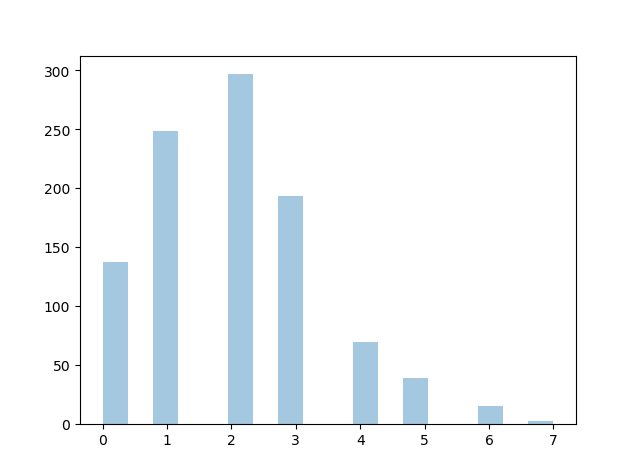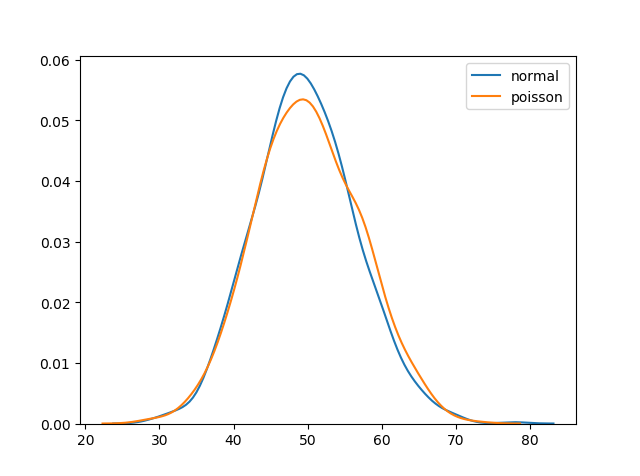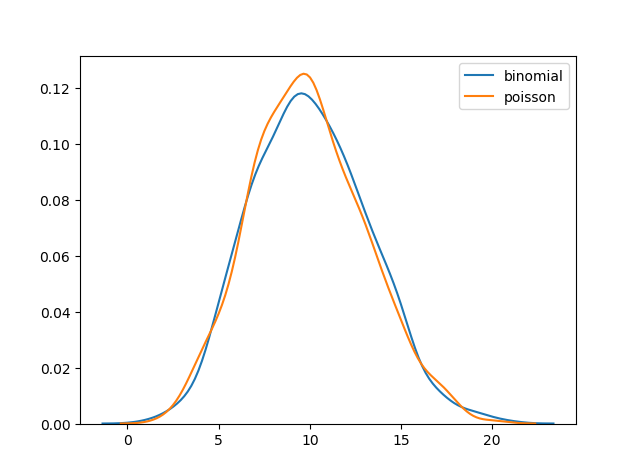# Poisson Distribution

## Poisson Distribution

Poisson Distribution is a Discrete Distribution.

It estimates how many times an event can happen in a specified time. e.g. If someone eats twice a day what is the probability he will eat thrice?

It has two parameters:

`lam` - rate or known number of occurrences e.g. 2 for above problem.

`size` - The shape of the returned array.

### Example

Generate a random 1x10 distribution for occurrence 2:

from numpy import random

x = random.poisson(lam=2, size=10)

print(x)
Try it Yourself »

## Visualization of Poisson Distribution

### Example

from numpy import random
import matplotlib.pyplot as plt
import seaborn as sns

sns.distplot(random.poisson(lam=2, size=1000), kde=False)

plt.show()

### ResultTry it Yourself »

## Difference Between Normal and Poisson Distribution

Normal distribution is continuous whereas poisson is discrete.

But we can see that similar to binomial for a large enough poisson distribution it will become similar to normal distribution with certain std dev and mean.

### Example

from numpy import random
import matplotlib.pyplot as plt
import seaborn as sns

sns.distplot(random.normal(loc=50, scale=7, size=1000), hist=False, label='normal')
sns.distplot(random.poisson(lam=50, size=1000), hist=False, label='poisson')

plt.show()

### ResultTry it Yourself »

## Difference Between Binomial and Poisson Distribution

Binomial distribution only has two possible outcomes, whereas poisson distribution can have unlimited possible outcomes.

But for very large `n` and near-zero `p` binomial distribution is near identical to poisson distribution such that `n * p` is nearly equal to `lam`.

### Example

from numpy import random
import matplotlib.pyplot as plt
import seaborn as sns

sns.distplot(random.binomial(n=1000, p=0.01, size=1000), hist=False, label='binomial')
sns.distplot(random.poisson(lam=10, size=1000), hist=False, label='poisson')

plt.show()

### ResultTry it Yourself »

W3Schools is optimized for learning and training. Examples might be simplified to improve reading and learning. Tutorials, references, and examples are constantly reviewed to avoid errors, but we cannot warrant full correctness of all content. While using W3Schools, you agree to have read and accepted our terms of use, cookie and privacy policy.